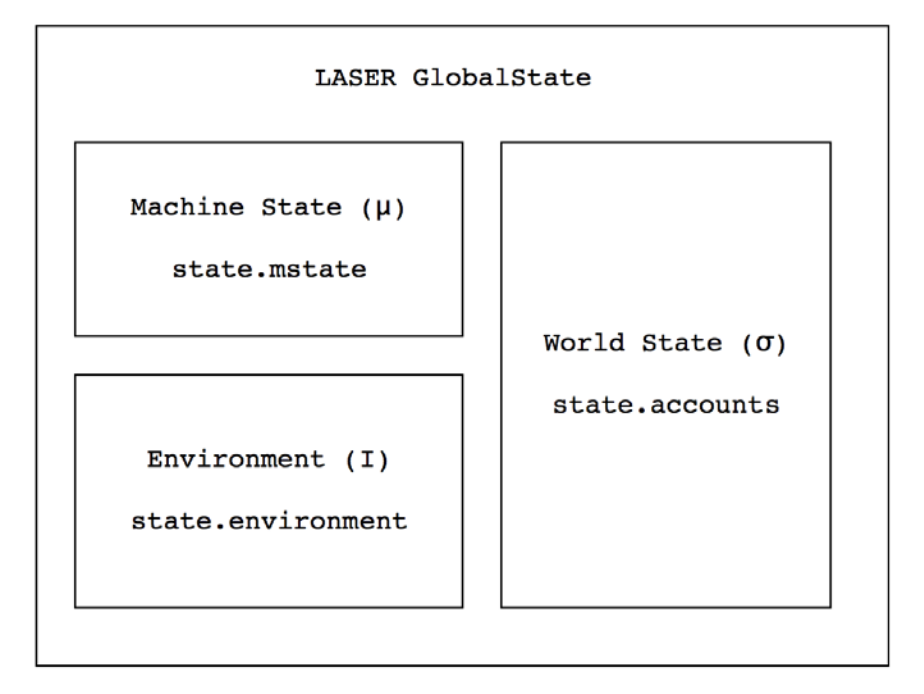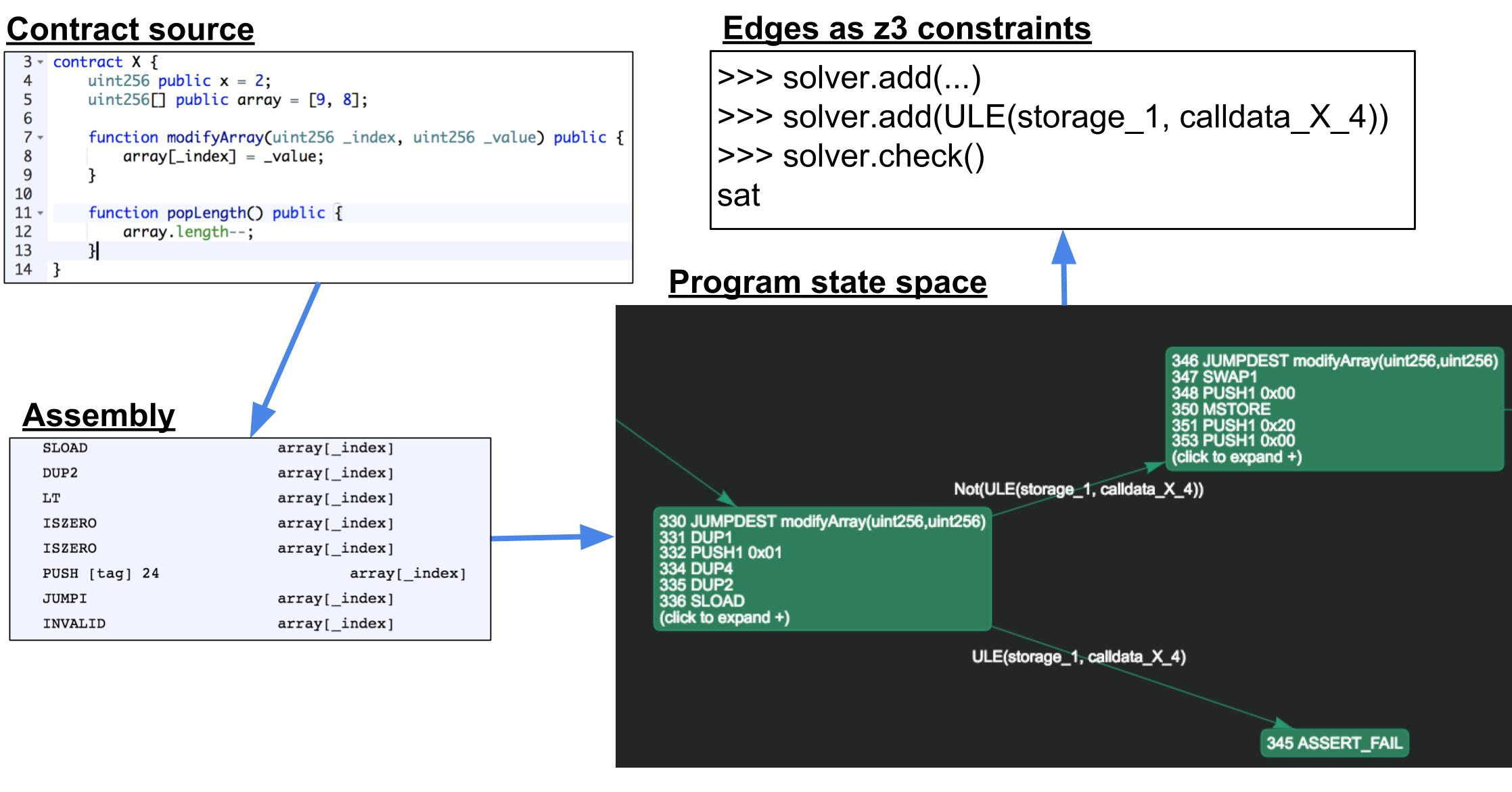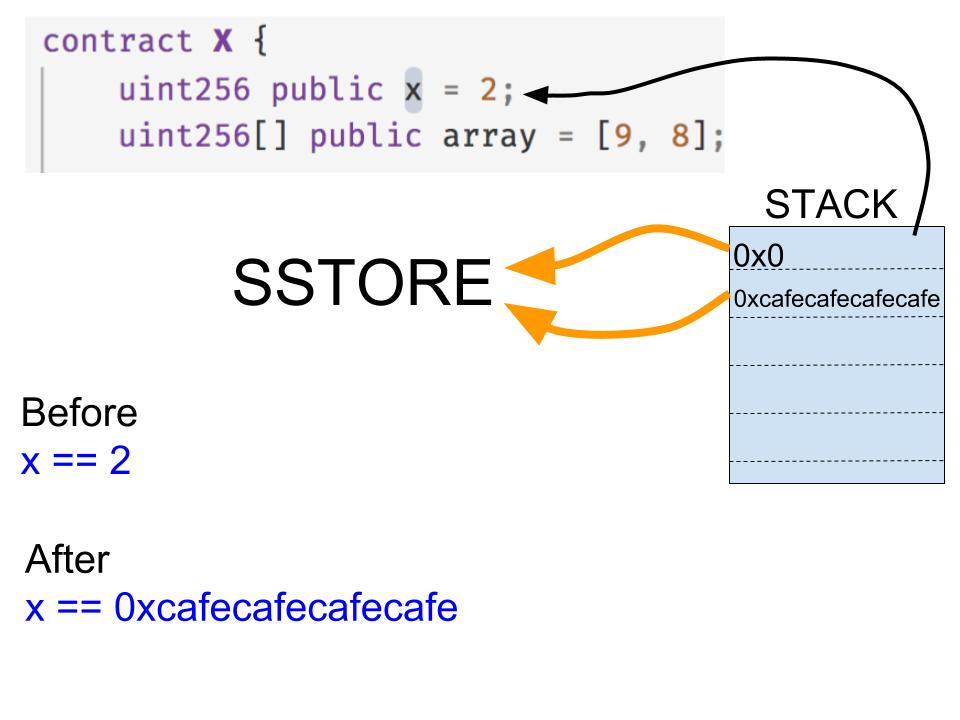2018.07.03

# Dynamic Analysis of Smart Contracts Using Mythril

Pocket

こんにちは、次世代システム研究室のN.M.です。

To dynamically analyze smart contracts Mythril uses an Ethereum Virtual Machine implemented in Python called Laser.

Mythril also makes use of Microsoft’s Z3, for verifying smart contracts.

Find out how Mythril uses Laser and Z3 to do formal verification on smart contracts.

Then use that knowledge to make use of Mythril’s API.

## Introducing Laser

Laser is Mythril’s EVM implemented in Python. It holds the following states,

### World State (σ)

The world state is a mapping of addresses to accounts. Each account holds balance, storage, nonce etc.

### Environment State (I)

Environment state is information about the transaction currently executing such as sender address, value, data input and so on.

### Machine State (μ)

Machine state is the current state of the machine including program counter, stack and memory.## Introducing Z3

Z3 is an open source SAT Solver developed by Microsoft under MIT license. SAT stands for Satisfiability. So given constraints on parameters Z3 can find values for the parameters that satisfy the constraints. This is useful when we consider a smart contract can be expressed as constraints on input parameters.

For example below in the Python cli:
```>>> from z3 import *
>>> a = Int('a')
>>> b = Int('b')
>>> s = Solver()
>>> s.add(a + b == 4)
>>> s.check()
sat
>>> s.model()
[b = 3, a = 1]
```
We will be using the Python Z3 (z3py), so we  import that library. We first define two integer parameters a and b. We then create a solver and add constraints to it for a and b. We then check if the constraints are satisfiable. We then obtain values for a and b which satisfy our constraints.

It should be obvious that some mixes of constraints will not be satisfiable. For example the constraints a > b, b > c, c > a can’t be satisfied.
```>>> s = Solver()
>>> s.add(a > b, b > c, c > a)
>>> s.check()
unsat
```

## Combined, what do they give us?

For any block of code in a contract Laser and Z3 combined can tell us if the code is reachable and the input required to reach it.

This is useful for testing error conditions for example.

### Control Flow Graphs

Contract code is stored in the EVM as binary assembly.

This assembly can be run in Laser to produce a graph of all possible execution states. This is a Control Flow Graph (CFG).

A node on the CFG is some sequence of instructions (in assembly in our case) that execute in series and change the state.

The edges on the CFG are constraints that express the conditions required to reach the node.

A node on the CFG is only reachable if all the constraints along the path from root to node are solvable in Z3!

Look at the image below to get the general idea.1. It gets compiled into assembly
2. The assembly is run through Laser to produce a CFG
3. The edges on the CFG are checked in Z3 for satisfiability
…and this tells us if the node (in this case 345 ASSERT_FAIL) is reachable. If we have an ASSERT FAIL node that is reachable this tells us there is a problem with out code. Either an automatically generated assertion can fail (such as when get get an array index out of bounds exception) or an explicit assertion that we wrote can fail. Either way we need to inspect the code more closely for problems. In this case we see that the modifyArray method can take an arbitrary _index parameter value which can lead to an array index out of bounds exception.

### Using Asserts

Mythril uses such error nodes found in the CFG to alert us of potential problems in our code. One way of explicitly telling Mythril to check for problems is to write asserts in our code that specify constraints to check for:
```contract Assertions {

function assertion1(uint256 input) public {
assert(input * 4 < 1024);
}

function assertion2(uint256 input) public {
if (input > 256) {
throw;
}

assert(input * 4 <= 1024);
}
}
```
Now Mythril will Use the CFG to find the nodes where the asserts fail and pass the constraints required for failure to Z3. If these constraints are satisfiable Mythril warns us we have a failing assert.
```\$ myth -x Assertions.sol --verbose-report
==== Exception state ====
Type: Informational
Contract: Assertions
Function name: assertion1(uint256)
A reachable exception (opcode 0xfe) has been detected. This can be caused by type errors, division by zero, out-of-bounds array access, or assert violations. This is acceptable in most situations. Note however that `assert()` should only be used to check invariants. Use `require()` for regular input checking.
--------------------
In file: Assertions.sol:4

assert(input * 4 < 1024)

--------------------

DEBUGGING INFORMATION:

The exception is triggered under the following conditions:

calldata_Assertions_4: 0x200
calldata_Assertions_0: 0xe166a66300000000000000000000000000000000000000000000000000000000
calldatasize_Assertions: 0x4
callvalue: 0x0

--------------------
```
Above Mythril tells us that an assertion is failing, the debugging information can be hard to read at first, but it’s not so bad when you remember a couple of simple rules.
The values calldata_Assertions_[number] in Mythril’s output are the calldata values computed by the solver. Concatenating these values yields the transaction data that triggers the exception: calldata = 0xe166a6630000000000000000000000000000000000000000000000000000000000000200

calldata_Assertions_0 is the function called. This is derived by taking the first 32 bits of the hash of the function name. In this case we see it matches function assertion1(uint256)
```\$ myth --hash "assertion1(uint256)"
0xe166a663
```
We see that 0x200 (512) was passed as a parameter.

4 * 512 = 1024, causing the assertion to fail.

## Using the API

In addition to other well known exploits (integer overflow, underflow, reentry, etc) Mythril also checks for cases where assertions fail. These assertions may be implicit, caused by array index out of bounds, or explicit as written into the contract code.

But what happens when we want to catch a state that is not specified in one of the above cases? For example in the contract below can the variable x be overwritten?
```contract X {
uint256 public x = 2;
uint256[] public array = [9, 8];

function modifyArray(uint256 _index, uint256 _value) public {
array[_index] = _value;
}

function popLength() public {
array.length--;
}
}
```

### But first, some assembly:

To perform this kind of analysis we need to write our own python script and access the Mythril API.
But first it is necessary to take a slight detour into the mechanics of the EVM’s assembly language. In this case the SSTORE instruction is the only instruction capable of writing to storage and this is the instruction we are interested in.The SSTORE instruction takes two parameters from the top of the stack.

The first parameter (0x0 in our case) specifies the storage key location. A value of 0x0 means the first storage location in our contract where the value of x is stored. The second storage location 0x1 would be where the value of the array variable is stored and so on.

The second parameter (0xcafecafecafecafe in our case), is simply the value to be written in the store operation.

So before the SSTORE operation the value of x would be 2, and after the operation the value would be 0xcafecafecafecafe.

### The script

Because we want to check if such an operation takes place we can simply search the CFG programatically. The script to do so is shown below.

```from laser.ethereum import svm
from mythril.ether.soliditycontract import SolidityContract
from mythril.analysis import solver
from mythril.exceptions import UnsatError
from z3 import *
contract = SolidityContract("../contracts/X.sol")
print('contract: ', contract)
laser = svm.LaserEVM(accounts)
for k in laser.nodes:
node = laser.nodes[k]
for state in node.states:
if (state.get_current_instruction()['opcode'] == 'SSTORE'):
proposition = node.constraints
proposition.append(state.mstate.stack[-1] == 0)
try:
model = solver.get_model(proposition)
print("VIOLATION FOUND!")
for d in model.decls():
print("%s = 0x%x\n" % (d.name(), model[d].as_long()))
print("In line %s\n%s\n" % (codeinfo.lineno, codeinfo.code))
except UnsatError:
pass

print("Analysis completed.")
```
The above script instantiates a Solidity contract object for X.sol, our test contract.
Then the script invokes the Laser EVM sym_exec method on the contract to generate the CFG. Now that we have the CFG we can search the nodes for an SSTORE operation on storage location zero. So we add these search conditions to the existing Z3 constraints and try and solve for these new constraints. If we get an UnsatError we carry on. Otherwise if we have satisfied our constraints we have found a case where is overwritten! In this case we output the details of the source code information pertaining to the current instruction (SSTORE).

```\$ python scan_x.py
contract:
VIOLATION FOUND!
calldata_unknown_0 = 0x9399cce800000000000000000000000000000000000000000000000000000000

calldatasize_unknown = 0x4

callvalue = 0x0

In line 3
contract X {
uint256 public x = 2;
uint256[] public array = [9, 8];

function modifyArray(uint256 _index, uint256 _value) public {
array[_index] = _value;
}

function popLength() public {
array.length--;
}
}

VIOLATION FOUND!

calldata_unknown_0 = 0xfe4948c300000000000000000000000000000000000000000000000000000000

calldatasize_unknown = 0x4

callvalue = 0x0

In line 8
array[_index] = _value

Analysis completed.
```
The first violation found tells us that for storage index 1 (storage_1) set to 0x4ef1d2ad89edf8c4d91132028e8195cdf30bb4b5053d4f8cd260341d4805f30b we have a possible match. Storage index 1 is the location of array, which by actually holds the length of the array. So for a length of 0x4ef1d2ad89edf8c4d91132028e8195cdf30bb4b5053d4f8cd260341d4805f30b we have a possible violation. This doesn’t really tell us enough to be useful.

The next violation tells us that calling a function matching 0xfe4948c3 with parameter of 0x4ef1d2ad89edf8c4d91132028e8195cdf30bb4b5053d4f8cd260341d4805f30a will enable us to change the value of x.

Using Mythril to get the hash for modifyArray:
```\$ myth --hash "modifyArray(uint256,uint256)"
0xfe4948c3
```
We have a match. If we call this function with an index of 0x4ef1d2ad89edf8c4d91132028e8195cdf30bb4b5053d4f8cd260341d4805f30a we can overwrite x with the supplied _value. But one problem remains if we simply reference such a high value for our array we will surely get an array out of bounds exception. Looking back at the first violation we see the length was actually set to our desired index parameter plus one. This gives a clue.

Another clue is the popLength function. This has no check for integer overflow/underflow. So we can underflow by calling the function 3 times (the array starts at length 2). Then we will have a length equal to the maximum integer for uint256 which is 2 ** 256 = 0xffffffffffffffffffffffffffffffff.

Now we are free to call modifyArray(0x4ef1d2ad89edf8c4d91132028e8195cdf30bb4b5053d4f8cd260341d4805f30a, 0xcafecafecafe) which will have the effect of overwriting x with 0xcafecafecafe.

In this way we have used our knowledge of Laser, Z3 and Ethereum assembly to find an array overflow bug!